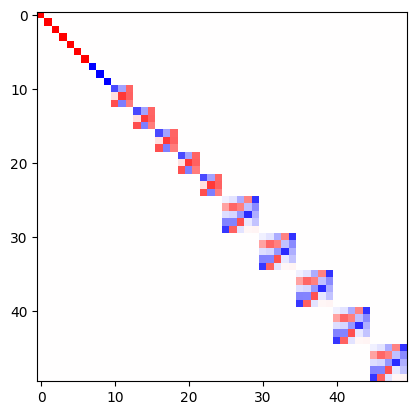# Irreducible representations

This page is a beginner introduction to the main object of `e3nn` library: `e3nn.o3.Irreps`. All the core component of `e3nn` can be found in `e3nn.o3`. `o3` stands for the group of 3d orthogonal matrices, which is equivalently the group of rotation and inversion.

```from e3nn.o3 import Irreps
```

An instance of `e3nn.o3.Irreps` describe how some data behave under rotation. The mathematical describtion of irreps can be found in the API Irreps.

```irreps = Irreps("1o")
irreps
```
```1x1o
```

`irreps` does not contain any data. Under the hood it is simply a tuple of made of other tuples and ints.

```# Tuple[Tuple[int, Tuple[int, int]]]
# ((multiplicity, (l, p)), ...)

print(len(irreps))
mul_ir = irreps  # a tuple

print(mul_ir)
print(len(mul_ir))
mul = mul_ir  # an int
ir = mul_ir  # another tuple

print(mul)

print(ir)
# print(len(ir))  ir is a tuple of 2 ints but __len__ has been disabled since it is always 2
l = ir
p = ir

print(l, p)
```
```1
1x1o
2
1
1o
1 -1
```

Our `irreps` means “transforms like a vector”. `irreps` is able to provide the matrix to transform the data under a rotation

```import torch
t = torch.tensor

# show the transformation matrix corresponding to the inversion
irreps.D_from_angles(alpha=t(0.0), beta=t(0.0), gamma=t(0.0), k=t(1))
```
```tensor([[-1., -0., -0.],
[-0., -1., -0.],
[-0., -0., -1.]])
```
```# a small rotation around the y axis
irreps.D_from_angles(alpha=t(0.1), beta=t(0.0), gamma=t(0.0), k=t(0))
```
```tensor([[ 0.9950,  0.0000,  0.0998],
[ 0.0000,  1.0000,  0.0000],
[-0.0998,  0.0000,  0.9950]])
```

In this example

```irreps = Irreps("7x0e + 3x0o + 5x1o + 5x2o")
```

the `irreps` tell us how 7 scalars, 3 pseudoscalars, 5 vectors and 5 odd representation of `l=2` transforms. They all transforms independently, this can be seen by visualizing the matrix

```from e3nn import o3
rot = -o3.rand_matrix()

D = irreps.D_from_matrix(rot)

import matplotlib.pyplot as plt
plt.imshow(D, cmap='bwr', vmin=-1, vmax=1);
```